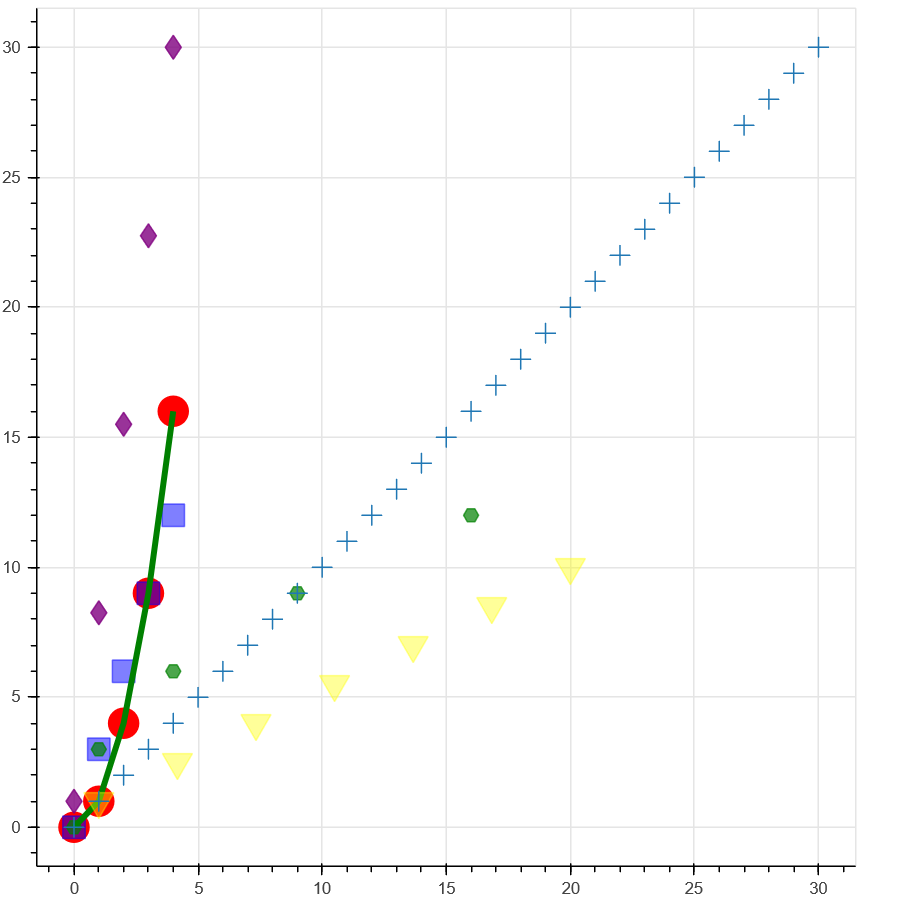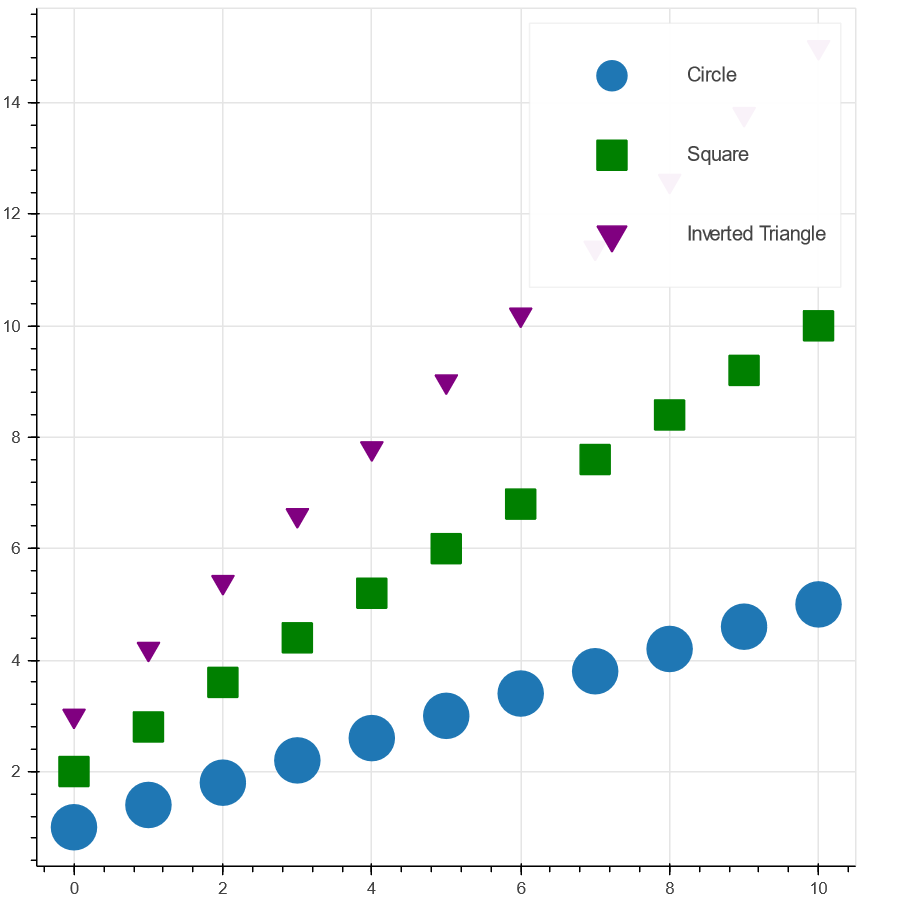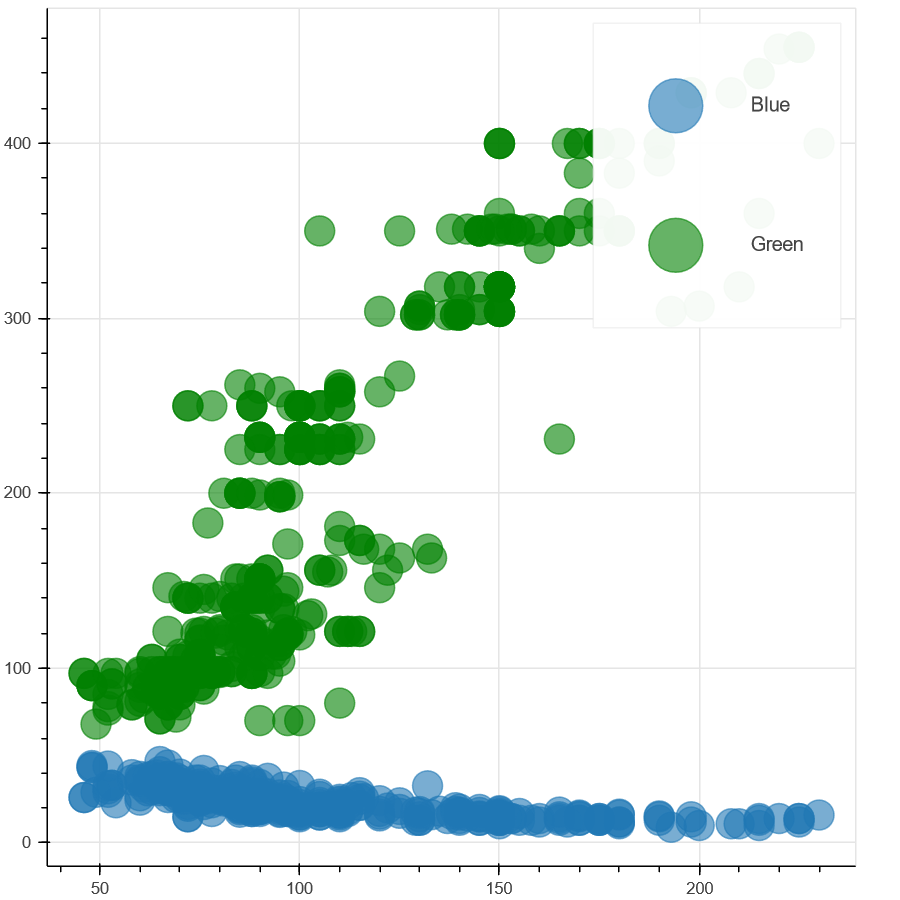GeeksforGeeks App
Open AppBrowser
Continue

# Create a plot with Multiple Glyphs using Python Bokeh

In this article, we will be learning about multiple glyphs and also about adding a legend in bokeh. Now bokeh provides us with a variety of glyphs that can be used to represent a point in a plot. Some glyphs are circle, square, asterik, inverted_triangle(), triangle() etc.

### Installation

This module does not come in-built with Python. To install it type the below command in the terminal.

`pip install bokeh`

## Multiple Glyphs in Bokeh

In this example, we will be exploring different types of glyphs in bokeh. Every glyph in the below implementation has different properties to identify themselves. So, lets us move to the code to understand the concept.

Code:

## Python3

 `# importing numpy package``import` `numpy as np` `# importing figure and show from``# bokeh.plotting module``from` `bokeh.plotting ``import` `figure, show` `# using numpy package to``# create a list of 5 numbers``x ``=` `np.arange(``5``)` `# y is stored with the square``# of the numbers in x``y ``=` `x``*``*``2` `# z is storing 3 times the value``# of the elements in x``z ``=` `x``*``3` `# storing 7 numbers in p between``# 1 to 20``p ``=` `np.linspace(``1``,``20``,``7``)` `# storing 7 numbers in q between``# 1 to 10``q ``=` `np.linspace(``1``, ``10``, ``7``)` `# storing 5 numbers in r between``# 1 to 30``r ``=` `np.linspace(``1``, ``30``, ``5``)` `# creating 31 elements in a list``a ``=` `np.arange(``31``)` `# creating an empty figure with specific plot``# width and height``fig ``=` `figure(plot_width ``=` `600` `, plot_height ``=` `600``)` `# plotting the points in the form of``# circular glyphs``fig.circle(x, y, color ``=` `"red"``, size ``=` `20``)` `# plotting the points in the form of``# square glyphs``fig.square(x, z, color ``=` `"blue"``, size ``=` `15``, alpha ``=` `0.5``)` `# plotting the points in the form of``# hex glyphs``fig.``hex``(y, z, color ``=` `"green"``, size ``=` `10``, alpha ``=` `0.7``)` `# drawing a line between the plotted points``fig.line(x, y, color ``=` `"green"``, line_width ``=` `4``)` `# plotting the points in the form of``# inverted triangle glyph``fig.inverted_triangle(p, q, color ``=` `"yellow"``, size ``=` `20``, alpha ``=` `0.4``)` `# plotting the points in the form of``# diamond glyphs``fig.diamond(x, r, color ``=` `"purple"``, size ``=` `16``, alpha ``=` `0.8``)` `# plotting the points in the form of``# cross glyphs` `fig.cross(a, a, size ``=` `14``)` `# showing the above plot``show(fig)`

Output:Explanation:

• At first, we are importing numpy, figure, and show packages from different modules.
• Now, we are creating a range of numbers using arrange and storing the list in x.
• Thereafter, we are also initializing different variables in order to plot the graph against each other.
• After initialization, we are creating an empty figure with plot width and height as 600.
• Since bokeh provides us with different types of glyphs, we are implementing some of them in this example with various colors and sizes of the points. The set of points with shape as circle has a color red and size as 20 whereas the set of points with shape as a hex has green color along with a size of 10 and the opacity of the color is 0.7(denoted by alpha).

## Multiple glyphs with bokeh legend

Now, lets us move to the next topic of this article and that is bokeh legend. Legend plays a very important role in bokeh plots. They help us to identify different types of glyphs that are used in a plot. Along with that, we can also change different properties of the box that can differentiate different glyphs.

Example 1:

In this example, we will be exploring multiple glyphs along with bokeh legend to identify them and also changing some properties of the box differentiating the plots. This is important because, in a plot with various types of glyphs, we need something to identify them and that is when bokeh legend plays its role. Lets us move to the code implementation now.

Code:

## Python3

 `# importing numpy as np``import` `numpy as np` `# importing figure and show from``# bokeh.plotting``from` `bokeh.plotting ``import` `figure, show` `# creating a list of numbers from 0-10``x ``=` `np.arange(``11``)` `# Creating square of the numbers and``# storing it in y``y ``=` `np.linspace(``1``,``5``,``11``)` `# Creating an array of random values in``# z``z ``=` `np.linspace(``0``,``2``,``11``)` `# Creating a plot with plot width and height``# as 600``p ``=` `figure(plot_height ``=` `600` `, plot_width ``=` `600``)` `# Plotting first line in the form of circle``p.circle(x, y, legend_label ``=` `"Circle"``, size ``=` `30``)` `# Creating first line in the form of square``p.square(x, y``*``2``, legend_label ``=` `"Square"``, size ``=` `20``, color ``=` `"green"``)` `# Creating forth line in the form of line``p.inverted_triangle(x, y``*``3``, legend_label ``=` `"Inverted Triangle"``,``                    ``size ``=` `15``, color ``=` `"purple"``)` `# Increasing the glyph height``p.legend.glyph_height ``=` `50` `# increasing the glyph width``p.legend.glyph_width ``=` `90` `# showing the above plot``show(p)`

Output:Explanation:

• We are creating three different variables with a set of values that are plotted against each other.
• We can see three plots, each of different glyphs. According to the code we have provided circular glyphs with size 30, square glyphs with size 20, and inverted triangle glyphs with size 15. Also, all the glyphs are of different colors too.

The main concept that lies in this example is, we have provided labels for each of the plots in the graph, and using legend in bokeh, we are changing the properties of the glyphs shown in the box in the top right corner(which is by default).

Example 2:

In the last example, we will be importing a dataset to our code. After that we will be plotting different graphs and using bokeh legend, we will be changing the label size and glyph size respectively. Let us move to the implementation.

Code:

## Python3

 `# importing autompg dataset from bokeh.sampledata.autompg``from` `bokeh.sampledata.autompg ``import` `autompg` `# importing columndatasource package from``# bokeh.models module``from` `bokeh.models ``import` `ColumnDataSource` `# importing figure and show from``# bokeh.plotting module to plot the figure``from` `bokeh.plotting ``import` `figure, show` `# provides data to the glyphs of the plot``source ``=` `ColumnDataSource(autompg)` `# Creating an empty figure``p ``=` `figure(plot_height ``=` `600``, plot_width ``=` `600``)` `# Creating circular glyphs with points plotted from``# columns taken from auto-mpg dataset``p.circle(x ``=` `'hp'``, y ``=` `'mpg'``, size ``=` `20``,``         ``alpha ``=` `0.6``, source ``=` `autompg ,``         ``legend_label ``=` `"Blue"``)` `# Creating circular glyphs with points plotted from``# columns taken from auto-mpg dataset  with different colors``p.circle(x ``=` `'hp'``, y ``=` `'displ'``, size ``=` `20``,``         ``alpha ``=` `0.6``, color ``=` `"green"` `, source ``=` `autompg,``         ``legend_label ``=` `"Green"``)` `# Adjusting the label height using legend``p.legend.label_height ``=` `50` `# Adjusting the label_width using legend``p.legend.label_width ``=` `50` `# Adjusting the glyph width using legend``p.legend.glyph_width ``=` `90` `# Adjusting the glyph height using legend``p.legend.glyph_height ``=` `90` `# Showing the above plot``show(p)`

Output:Explanation:

• Along with importing all the packages which we have imported earlier, this time we are importing a new dataset auto-mpg. Along with that, we are also importing the ColumnDataSource package.
• With ColumnDataSouce package we are creating it manually which helps us to share data between multiple plots and widgets.
• After that, we are plotting data taken from auto-mpg data-set against each other.
• Then using legend property of bokeh (figure.legend.label_width,figure.legend.glyph_width,figure.legend.label_height,figure.legend.glyph_height) we are changing the size of the labels and glyphs used to identify plots in the graph.

My Personal Notes arrow_drop_up